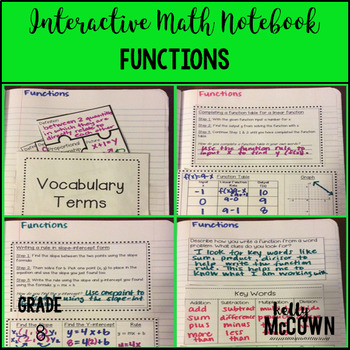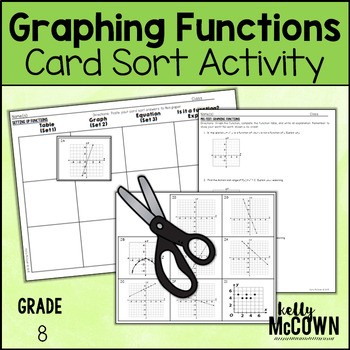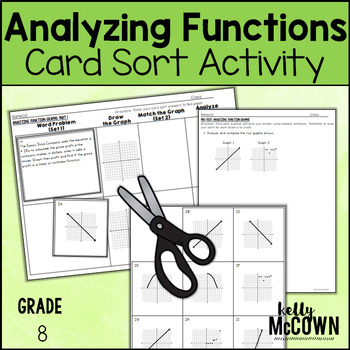## Friday, October 28, 2016

One skill that students need to master in eighth grade is functions. In sixth and seventh grade students lay the foundations for equations and functions. Now that they are in eighth grade they are using the equation skills from sixth and seventh grade. Students often make mistakes with setting up the function table, making connections between the function table and graph or function table and equation. These are simple mistakes that you can prevent with practice.

I have listed here activities to review and practice Functions. I hope these help jump start your lesson planning. The key to getting your students involved in the learning process is to include interactive, visual, engaging activities for them to actively participate in.This math station activity is intended to help students understand how to graph proportional relationships, understand that a function is a rule that assigns one input to one output exactly, compare properties of two functions, interpret the equation y = mx + b, construct a function, determine the rate of change of a function, and describe the functional relationship between two quantities.

Included are:
-6 different stations to engage students
-Teacher facilitated activity for 60-90 minutes of classroom time
-Stations include:
1. Vocabulary
3. Practice of Functions
5. Real World applications of Functions
6. Word problems of Functions
-Student Station Guide helps students record their answersThese interactive notebook activities are intended to help students understand how to graph proportional relationships, understand that a function is a rule that assigns one input to one output exactly, compare properties of two functions, interpret the equation y = mx + b, construct a function, determine the rate of change of a function, and describe the functional relationship between two quantities.

Included are:
-4 different Functions activities to engage students
-Teacher facilitated activity for 60-90 minutes of classroom time
-Activities include: Vocabulary, Completing a function table and graph from a linear function rule, Writing a rule from two points using slope-intercept form, and Writing a rule, completing a function table, and drawing a graph from a function word problem.
-Examples of Completed Interactive Math Notebook Activities are includedThis lesson unit is intended to help you assess how well students are able to assess and graph functions in tables, graphs, and equations.

Included in this lesson are:
-card sort activity with concept development of functions
-one extension activity for students to deepen their conception of functions
-answer keys for all the assessments and activities
-how the lesson is tied to Common core and mathematical practicesThis lesson unit is intended to help you assess how well students are able to describe qualitatively the functional relationship between two quantities by analyzing a graph (e.g., where the function is increasing or decreasing, linear or nonlinear). Sketch a graph that exhibits the qualitative features of a function that has been described verbally.

Included in this lesson are: